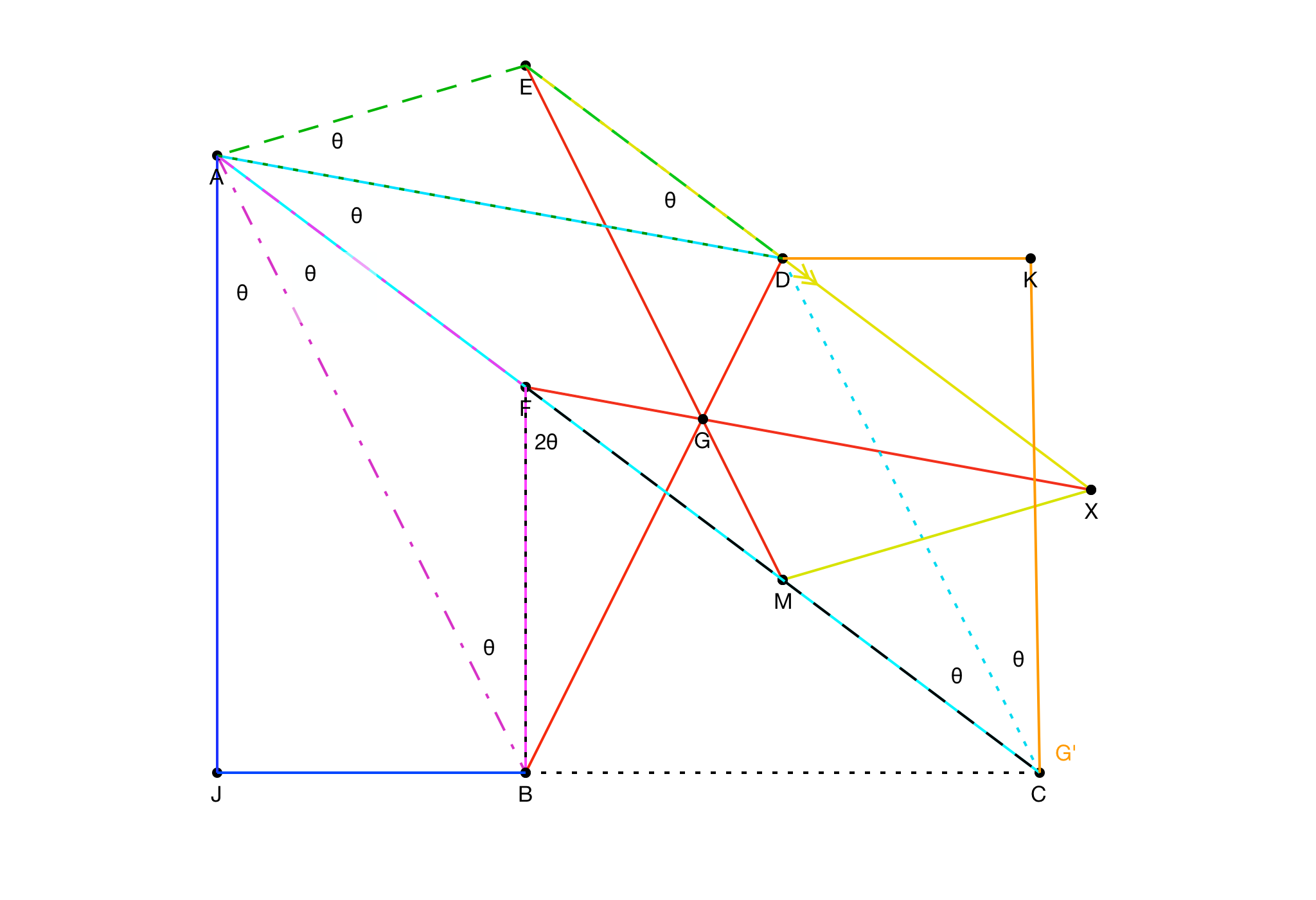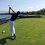# IMO Day 1 Problem 1: Vector/trig bash

I almost forgot how fun challenging math was until my plane ride last week where I solved IMO problem 1. Then I fell right back in love with math again. Anyway, let's begin. (Please excuse my notations and terminology, I'm a bit rusty on that).

## Problem:

Triangle $BCF$ has a right angle at $B$. Let $A$ be the point on line $CF$ such that $FA = FB$ and $F$ lies between $A$ and $C$. Point $D$ is chosen so that $DA = DC$ and $AC$ is the bisector of $\angle{DAB}$. Point $E$ is chosen so that $EA = ED$ and $AD$ is the bisector of $\angle{EAC}$. Let $M$ be the midpoint of $CF$. Let $X$ be the point such that $AMXE$ is a parallelogram. Prove that $BD$, $FX$, and $ME$ are concurrent.

## SolutionLet $\overline{BC}=a,~\text{and}~\overline{FB}=bi$

We are given

\boxed{\begin{aligned} C &= \langle a,0 \rangle\\ B &= \langle 0,0 \rangle\\ M &= \langle \frac{a}{2},\frac{b}{2} \rangle \\ F &= \langle 0,b \rangle \end{aligned}}

Now let angle $\angle \text{JAB}=\theta$ and the rest are labeled in the picture for you. (IGNORE POINT G). Also, define $\overline{CF}=\sqrt{a^2+b^2}=r$.

Now define $P_x$ as the $x$-coordinate of point $P$ and define $P_y$ similarly. Segments $\overline{BF}$ and $\overline{FA}$ are both length $b$. Thus $C_x\cdot \dfrac{b}{r}=A_x$ and $F_y\cdot \dfrac{b+r}{r}=A_y$.

Some arithmetic yields $\boxed{A= \langle -\frac{ab}{r},\frac{b^2}{r}+b \rangle}$

Next, it is obvious that since $\overline{CA}=b+r$, segment $\overline{DC}=\overline{AD}=\dfrac{b+r}{2\cos(\theta)}$.

And it follows

$D=\vec{C}+\frac{b+r}{2\cos(\theta)}\cdot \text{cis}\left(\dfrac{\pi}{2}\right)$

$D= \langle a-\frac{b+r}{2}\tan(\theta), \frac{b+r}{2} \rangle$

Similar triangles tells us that $\overline{JB}=\frac{ab}{r}$ and point $A$ tells us that $\overline{JA}=\frac{b^2}{r}+b$.

Thus the picture shows us that $\tan{\theta}=\frac{a}{b+r}$

Amazingly, this means that point $D$ is directly over point $M$ with coordinates $\boxed{D= \langle \frac{a}{2}, \frac{b+r}{2} \rangle}$

Next, it is apparent that $\overline{AE}=\overline{ED}=\dfrac{\overline{AD}}{2\cos(\theta)}=\dfrac{b+r}{4\cos^2(\theta)}$.

Pythagorean theorem tells us that $\overline{AB}=b\sqrt{2+\frac{2b}{r}}$. Unbelievably, this is pretty much the only root we have to deal with besides $r$ in all of this problem. And this root goes away immediately. Props to the maker of this beautiful problem.

From $\triangle ABJ$ we know what $\cos^2(\theta)$ is and thus $\overline{AE}=\dfrac{b+r}{4\cos^2(\theta)}=\dfrac{r}{2}$.

Thus $E=\vec{A}+\text{cis}\left(\frac{3\pi}{2}+\frac{r}{2}\right)$

Not surprisingly, everything cancels once again and we are left with

$\boxed{E= \langle 0,b+\frac{r}{2} \rangle}$

Finally,

$\vec{X} =\vec{M}-\vec{A}+\vec{E}$

$\boxed{X =\langle \frac{a}{2}+\frac{ab}{r}, \frac{b+r}{2} -\frac{b^2}{r} \rangle}$

We now have

\boxed{\begin{aligned} C &= \langle a,0 \rangle\\ B &= \langle 0,0 \rangle\\ M &= \langle \frac{a}{2},\frac{b}{2} \rangle \\ F &= \langle 0,b \rangle \\ A &= \langle -\frac{ab}{r},\frac{b^2}{r}+b \rangle \\ D &= \langle \frac{a}{2}, \frac{b+r}{2} \rangle \\ E &= \langle 0,b+\frac{r}{2} \rangle \\ X &=\langle \frac{a}{2}+\frac{ab}{r}, \frac{b+r}{2} -\frac{b^2}{r} \rangle \end{aligned}}

I didn't take the time to actually work out the lines in variable form. But plugging in values for a and b show that the three lines are concurrent.

I unfortunately lost the paper that I had the coordinates of intersection on. But the coordinates are sooo pretty, no radicals, just simple, elegant fractions.Note by Trevor Arashiro
4 years, 12 months ago

This discussion board is a place to discuss our Daily Challenges and the math and science related to those challenges. Explanations are more than just a solution — they should explain the steps and thinking strategies that you used to obtain the solution. Comments should further the discussion of math and science.

When posting on Brilliant:

• Use the emojis to react to an explanation, whether you're congratulating a job well done , or just really confused .
• Ask specific questions about the challenge or the steps in somebody's explanation. Well-posed questions can add a lot to the discussion, but posting "I don't understand!" doesn't help anyone.
• Try to contribute something new to the discussion, whether it is an extension, generalization or other idea related to the challenge.

MarkdownAppears as
*italics* or _italics_ italics
**bold** or __bold__ bold
- bulleted- list
• bulleted
• list
1. numbered2. list
1. numbered
2. list
Note: you must add a full line of space before and after lists for them to show up correctly
paragraph 1paragraph 2

paragraph 1

paragraph 2

[example link](https://brilliant.org)example link
> This is a quote
This is a quote
    # I indented these lines
# 4 spaces, and now they show
# up as a code block.

print "hello world"
# I indented these lines
# 4 spaces, and now they show
# up as a code block.

print "hello world"
MathAppears as
Remember to wrap math in $$ ... $$ or $ ... $ to ensure proper formatting.
2 \times 3 $2 \times 3$
2^{34} $2^{34}$
a_{i-1} $a_{i-1}$
\frac{2}{3} $\frac{2}{3}$
\sqrt{2} $\sqrt{2}$
\sum_{i=1}^3 $\sum_{i=1}^3$
\sin \theta $\sin \theta$
\boxed{123} $\boxed{123}$